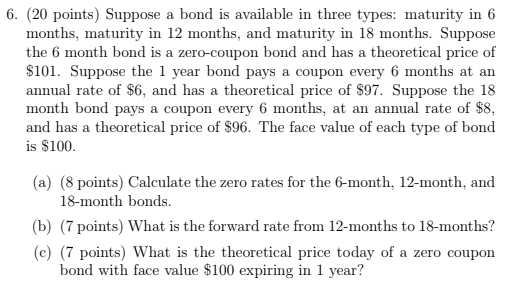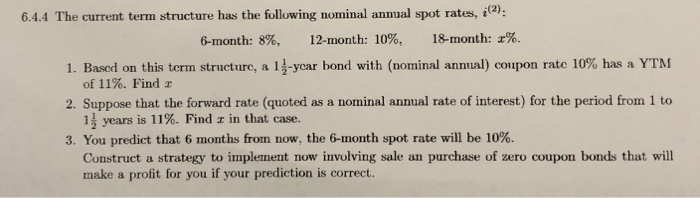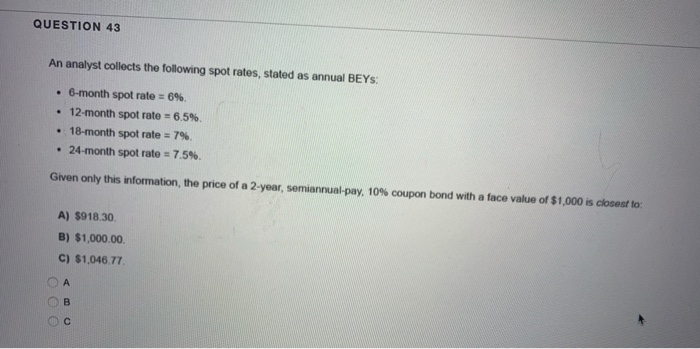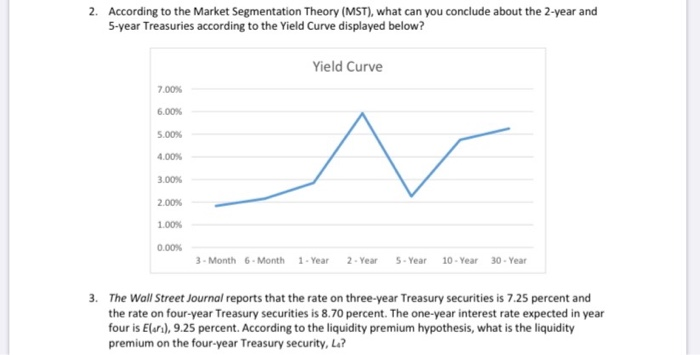Search Results
• #### Consider the following spot rate curve: 6-month spot rate: 6%. 12-month spot rate: 11%. 18-month spot...

Consider the following spot rate curve: 6-month spot rate: 6%. 12-month spot rate: 11%. 18-month spot rate: 14%. What is the forward rate for a 6-month zero coupon bond issued one year from today? Equivalently, the question asks for f12, where 1 time period consists of 6 months. Remember, like spot rates, forward rates are expressed as bond-equivalent yields.

• #### 1) Consider a 10-year bond trading at \$1150 today. The bond has a face value of...

1) Consider a 10-year bond trading at \$1150 today. The bond has a face value of \$1,000, and has a coupon rate of 8%. Coupons are paid semiannually, and the next coupon payment is exactly 6 months from now. What is the bond's yield to maturity? 2)A coupon-paying bond is trading below par. How does the bond's YTM compare to...

• #### 6. (20 points) Suppose months, maturity in 12 months, and maturity in 18 months. Suppose the 6 month bond is a zero-cou...6. (20 points) Suppose months, maturity in 12 months, and maturity in 18 months. Suppose the 6 month bond is a zero-coupon bond and has a theoretical price of \$101. Suppose the 1 year bond pays a coupon every 6 months at an annual rate of \$6, and has a theoretical price of \$97. Suppose the 18 month bond pays...

• #### 6.4.4 The current term structure has the following nominal annual spot rates, i2) 18-month: % 12-month:...6.4.4 The current term structure has the following nominal annual spot rates, i2) 18-month: % 12-month: 10%, 6-month: 8%, 1. Based on this term structure, a 13-ycar bond with (nominal annual) coupon rate 10% has a YTM of 11%. Find x 2. Suppose that the forward rate (quoted as a nominal annual rate of interest) for the period from 1...

• #### QUESTION 43 An analyst collects the following spot rates, stated as annual BEYS: • 6-month spot...QUESTION 43 An analyst collects the following spot rates, stated as annual BEYS: • 6-month spot rate = 6%. • 12-month spot rate = 6.5%. • 18-month spot rate = 7% • 24-month spot rate = 7.5%. Given only this information, the price of a 2-year, semiannual-pay, 10% coupon bond with a face value of \$1,000 is closest to: A)...

• #### All interest and inflation rates are stated as annual rates. Unbiased forward rate (forward expectation parity)...

All interest and inflation rates are stated as annual rates. Unbiased forward rate (forward expectation parity) 1. If the spot market exchange rate for the euro is 1.1427 and the 6-month forward quote is 178, what is the expected exchange rate for the euro in six months? 2. If the spot market exchange rate for the Hong Kong dollar is...

• #### What does the current Treasury Yield Curve look like today? What does this say about the...

What does the current Treasury Yield Curve look like today? What does this say about the expectation of interest rates in the future? What does it say about inflation? How does it compare to the yield curve a month ago? A year ago? Now look at the yield curve from November 20, 2006. What was this curve predicting?

• #### solve question 1 and 2 please i mean 2 and 3 2. According to the Market...solve question 1 and 2 please i mean 2 and 3 2. According to the Market Segmentation Theory (MST), what can you conclude about the 2-year and 5-year Treasuries according to the Yield Curve displayed below? Yield Curve 7.00% 6.00% 5.00% 4.00% 3.00% 2.00% 1.00% 0.00% 3 - Month 6 Month 1-Year 2-Year 5 Year 10-Year 30-Year 3. The Wall...

• #### All interest and inflation rates are stated as annual rates. International Fisher effect 4. If the...

All interest and inflation rates are stated as annual rates. International Fisher effect 4. If the spot market exchange rate for the Haitian gourde is 783.961, the 1-year interest rate paid on Haitian government debt is 20.0%, and the 1-year interest rate on US government debt is 2.60%, what is the expected exchange rate for the gourde in one year?...

• #### The 6-month, 12-month, 18-month, and 24-month zero rates are 4%, 4.5%, 4.75%, and 5% with semiannual...

The 6-month, 12-month, 18-month, and 24-month zero rates are 4%, 4.5%, 4.75%, and 5% with semiannual compounding, respectively. (a) What are the rates with continuous compounding? (b) What is the forward rate for the six-month period beginning in 18 months? (c) What is the value of an FRA that promises to pay you 6% (with semiannual payment) on a principal...